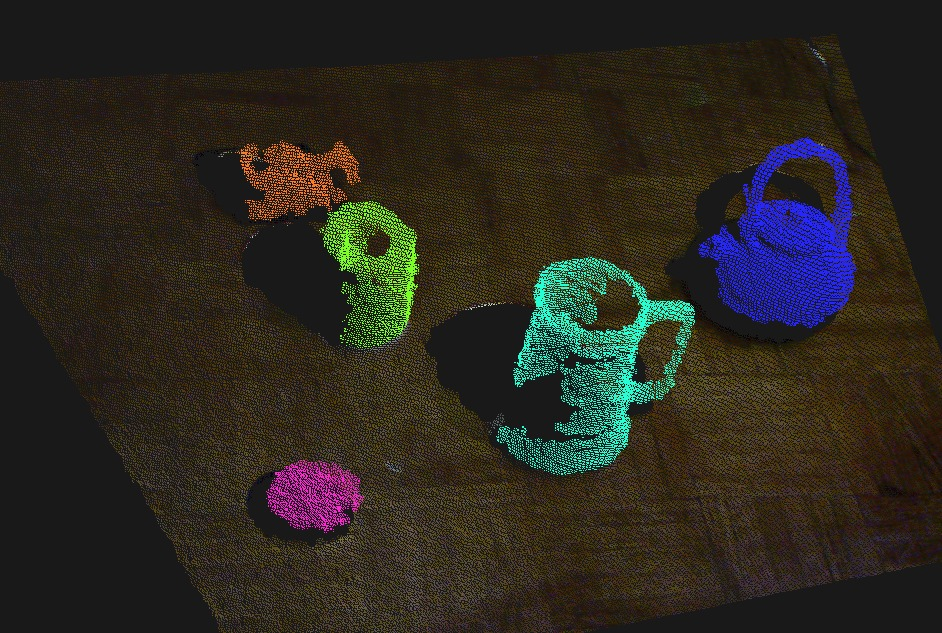#### Previous topic

Tabletop Segmentation

#### Next topic

Display a point cloud from a PCD file

# Segment the objects from the plane, color them¶colorize_clusters.py

```#!/usr/bin/env python

"""
This example shows how to extract points corresponding to objects on a table,
cluster them, colorize the clusters, and republish as a single cloud.

1) The example downsamples using a VoxelGrid before estimating
normals for the downsampled cloud.
2) These normals are then used for segmentation using RANSAC.
3) Segmentation produces a planar model to which all inliers are
projected so that a 2D convex hull can be created.
4) We then extract the indices of all points that are above the
plane formed by the convex hull.
5) This cloud is then clustered
6) The clusters are concatenated, with each having a unique color.

"""

import ecto
from ecto.opts import run_plasm, scheduler_options
from ecto_openni import Capture
from ecto_pcl import *

# capture from kinect and downsample
device = Capture('device')
cloud_generator = NiConverter('cloud_generator')
voxel_grid = VoxelGrid("voxel_grid", leaf_size=0.05)

graph = [device[:] >> cloud_generator[:],
cloud_generator[:] >> voxel_grid[:]
]

# estimate normals, segment and find convex hull
planar_segmentation = SACSegmentationFromNormals("planar_segmentation",
model_type=SACMODEL_NORMAL_PLANE,
eps_angle=0.09, distance_threshold=0.1)
project_inliers = ProjectInliers("project_inliers", model_type=SACMODEL_NORMAL_PLANE)
nan_filter = PassThrough('nan_removal')
convex_hull = ConvexHull("convex_hull")

graph += [voxel_grid[:] >> normals[:],
voxel_grid[:] >> planar_segmentation["input"],
normals[:] >> planar_segmentation["normals"],
voxel_grid[:] >> project_inliers["input"],
planar_segmentation["model"] >> project_inliers["model"],
project_inliers[:] >> nan_filter[:],
nan_filter[:] >> convex_hull['input']
]

# extract stuff on table from original high-res cloud, find clusters, colorize, merge and show in viewer
extract_stuff = ExtractPolygonalPrismData("extract_stuff", height_min=0.01, height_max=0.2)
extract_indices = ExtractIndices("extract_indices", negative=False)
extract_clusters = EuclideanClusterExtraction("extract_clusters", min_cluster_size=50, cluster_tolerance=0.005)
colorize = ColorizeClusters("colorize")
merge = MergeClouds("merge")
viewer = CloudViewer("viewer", window_name="Clouds!")

graph += [cloud_generator[:] >> extract_stuff["input"],
convex_hull[:] >> extract_stuff["planar_hull"],
extract_stuff[:] >> extract_indices["indices"],
cloud_generator[:] >> extract_indices["input"],
extract_indices[:] >> extract_clusters['input'],
extract_clusters[:] >> colorize["clusters"],
extract_indices[:] >> colorize["input"],
cloud_generator[:] >> merge["input"],
colorize[:] >> merge["input2"],
colorize[:] >> viewer[:]
]

plasm = ecto.Plasm()
plasm.connect(graph)

if __name__ == "__main__":
from ecto.opts import doit
doit(plasm, description='Execute tabletop segmentation and colorize clusters.')
```